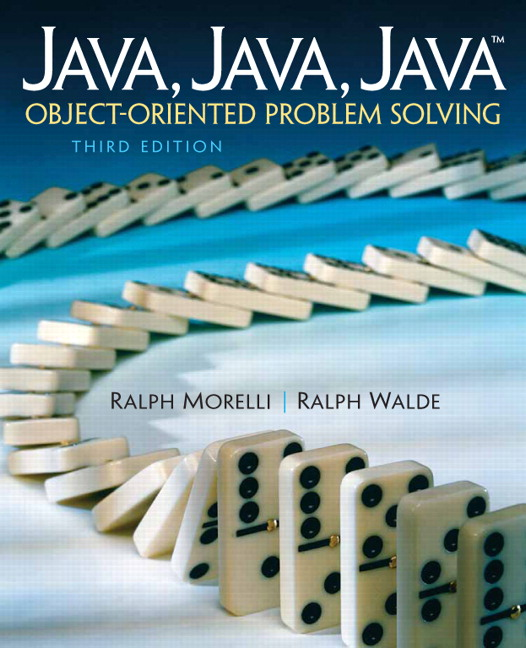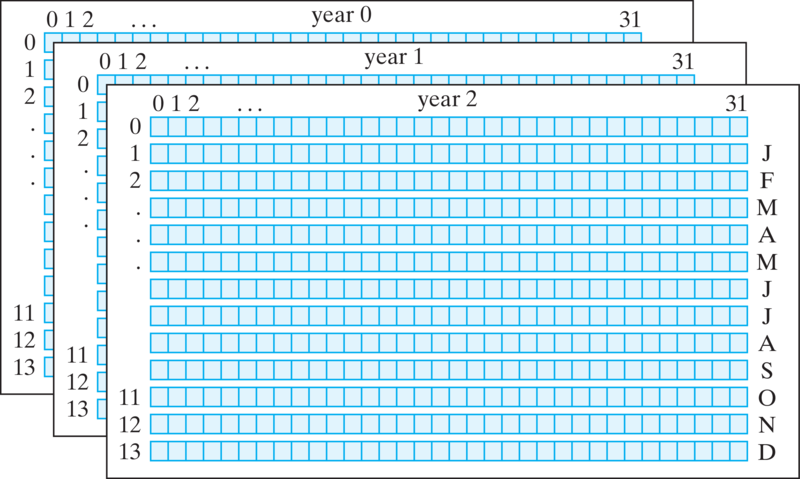# Java, Java, Java: Object-Oriented Problem Solving, 2022E

## Section9.8Multidimensional Arrays (Optional)

Java doesn’t limit arrays to just two dimensions. For example, suppose we decide to extend our rainfall survey to cover a ten-year period. For each year we now need a two-dimensional array. This results in a three-dimensional array consisting of an array of years, each of which contains an array of months, each of which contains an array of days:
final int NYEARS = 10;
final int NMONTHS = 13;
final int NDAYS = 32;
double rainfall[][] = new double[NYEARS][NMONTHS][NDAYS];

Following the design convention of not using the 0 month and 0 days, we end up with a $$10 \;\times\; 13 \;\times\; 32$$ array. Note the use of final variables to represent the size of each dimension of the array. This helps to make the program more readable.
In Figure 9.8.1, each year of the rainfall data is represented as a separate page. On each page, there is a two-dimensional table that consists of 12 rows (1 per month) and 31 columns (1 per day).Figure 9.8.1. Three-dimensional data might be viewed as a collection of pages, each of which contains a two-dimensional table.
You might imagine that our study could be extended to cover rainfall data from a number of different cities. That would result in a four-dimensional array, with the first dimension now being the city. Of course, for this to work, cities would have to be represented by integers, because array subscripts must be integers.
As you might expect, algorithms for processing each element in a three-dimensional table would require a three-level nested loop. For example, the following algorithm would be used to initialize all elements of our three-dimensional rainfall array:
for (int year = 0; year < rainfall.length; year++)
for (int month = 0; month < rainfall[year].length; month++)
for (int day = 0; day < rainfall[year][month].length; day++)
rainfall[year][month][day] = 0.0;

Note again the proper use of the length attribute for each of the three dimensions of the array. In the outer loop, rainfall.length, we’re referring to the number of years. In the middle loop, rainfall[year].length, we’re referring to number of months within a given year. In the inner loop, rainfall[year][month].length, we’re referring to the number of days within a month.
If we added a fourth dimension to our array and wanted to extend this algorithm to initialize it, we would simply embed the three-level loop within another for loop that would iterate over each city.

### Subsection9.8.1Array Initializers

It is possible to use an initializer with a multidimensional array. For instance, the following examples create several small arrays and initialize their elements:
int a[][] = {{1,2,3}, {4,5,6}};
char c[][] = {{'a','b'}, {'c','d'}};
double d[][][] =
{{1.0,2.0,3.0}, {4.0,5.0}, {6.0,7.0,8.0,9.0}};

The first of these declarations creates a $$2\; \times \;3$$ array of integers. The second example creates a $$2\; \times\; 2$$ array of characters, and the third example creates an array of double consisting of three rows, each of which has a different number of elements. The first row contains three elements, the second contains two elements, and the last row contains four elements. As this last example shows, the rows in a multidimensional array don’t all have to have the same length.
Using initializers, as in these examples, is feasible only for relatively small arrays. To see why, just imagine what the initializer expression would be for our three-dimensional rainfall array. It would require $$4,160 \;=\; 10 \; \times \; 13 \; \times \; 32$$ zeroes, separated by commas!